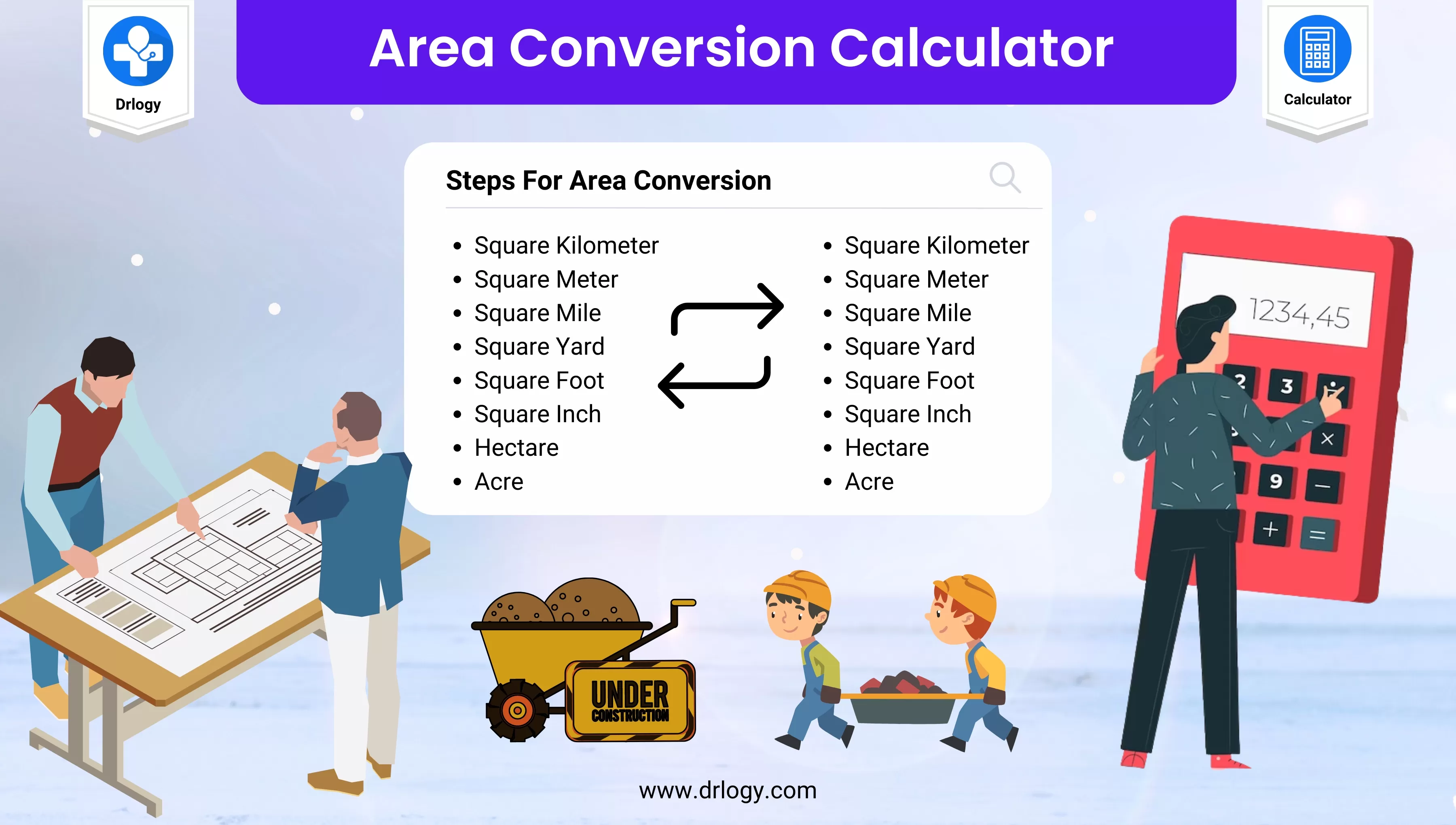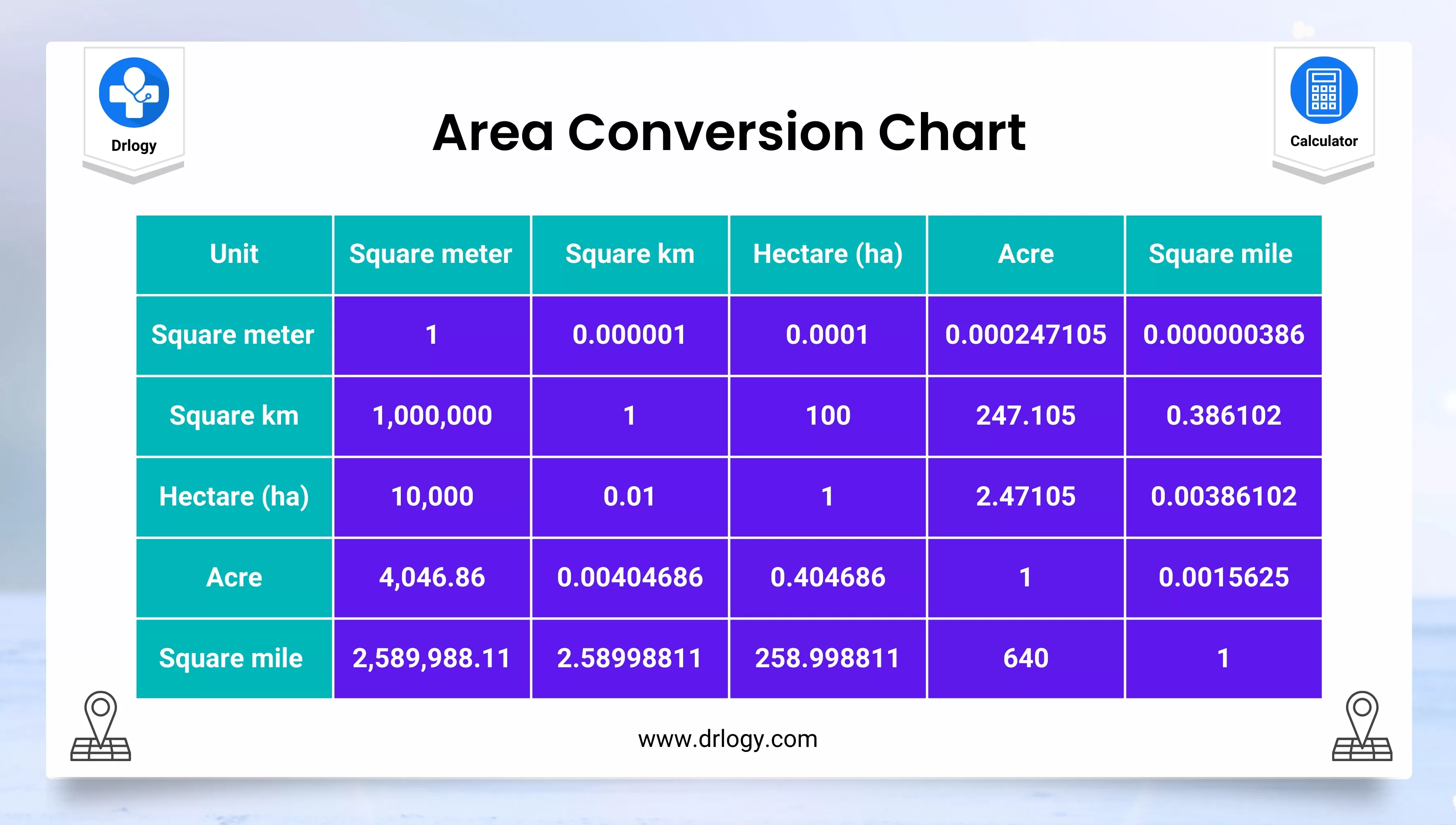• Health
• Pet
• Unit Conversion
• Medical# Area Conversion Calculator Units

## Area Conversion Calculator

Result : =
keyboard_arrow_down
=
keyboard_arrow_down

## Area Conversion Calculator

• An area conversion calculator is a tool that allows you to convert measurements of area from one unit to another.
• Some common units of area measurement include square meters, square feet, square miles, hectares, acres, and many more.
• Using an area conversion calculator can be helpful in a variety of situations, such as when you need to calculate the area of a room in a different unit than the one it was measured in, or when you need to convert land area measurements for real estate or construction purposes.
• When using an area conversion calculator, it's important to make sure you understand the units you are working with and the conversion factors between them.
• Some conversion factors may be straightforward, such as the fact that 1 square meter is equal to 10.764 square feet. However, others may be more complex, such as the conversion factor between hectares and acres.
• Many area conversion calculators are available online for free, and some are even built into calculator apps on smartphones and other devices.
• These calculators may allow you to convert between multiple units of area at once, or they may focus on converting between just a few commonly used units.

## Steps For Area Conversion Calculator

Here are the steps to use an area conversion calculator:

1. Determine the starting unit of measurement for the area you want to convert.
2. Determine the desired unit of measurement for the converted area.
3. Find an area conversion calculator online or using a calculator app.
4. Enter the value of the area you want to convert in the starting unit of measurement.
5. Select the starting unit of measurement from the calculator's drop-down menu or options.
6. Select the desired unit of measurement from the calculator's drop-down menu or options.
7. Review the result of the conversion in the desired unit of measurement.
8. If needed, repeat the process with additional area conversions.

• It's important to double-check that you have entered the correct units of measurement into the calculator and that you have selected the appropriate conversion factors.
• Some calculators may provide multiple options for the same unit of measurement, such as square feet versus square inches, so be sure to select the one you need.
• Additionally, be aware that some area conversion calculators may round or truncate the result, so it may be necessary to round to the desired level of precision manually.

## Area Conversion Calculator Chart

Here is a chart showing common units of area measurement and their conversion factors:

Unit of Measurement Abbreviation Conversion Factor
Square meter 1
Square centimeter cm² 0.0001
Square millimeter mm² 0.000001
Hectare ha 10,000
Square kilometer km² 1,000,000
Square inch in² 0.00064516
Square foot ft² 0.09290304
Square yard yd² 0.83612736
Acre ac 4046.8564224
Square mile mi² 2,589,988.110336

To use this chart, multiply the value of the area you want to convert by the conversion factor for the desired unit of measurement. For example, to convert 100 square meters to square feet, you would multiply 100 by 10.764, since 1 square meter is equal to 10.764 square feet. The result would be 1076.4 square feet.

## Area Conversion Calculator Formula

Here is a table showing the conversion formula and factors for common units of area measurement:

Unit of Measurement Conversion Formula Conversion Factor
Square meter (m²) 1m² = 1m² 1
Square centimeter (cm²) 1m² = 10,000cm² 0.0001
Square millimeter (mm²) 1m² = 1,000,000mm² 0.000001
Hectare (ha) 1ha = 10,000m² 10,000
Square kilometer (km²) 1km² = 1,000,000m² 1,000,000
Square inch (in²) 1m² = 1550.0031in² 0.00064516
Square foot (ft²) 1m² = 10.7639104ft² 0.09290304
Square yard (yd²) 1m² = 1.19599005yd² 0.83612736
Acre (ac) 1ac = 4046.85642m² 4046.85642
Square mile (mi²) 1mi² = 2,589,988.11034m² 2,589,988.11034

• To use this table, you can use the conversion formula and the conversion factor to convert the area from one unit of measurement to another.
• For example, to convert 100 square meters to square feet, you can use the conversion formula 1m² = 10.7639104ft², and multiply the area value (100) by the conversion factor (10.7639104). The result would be 1076.39104 square feet.## Area Conversion Calculator Table

Here is a table showing the conversion factors for some common units of area measurement:

From / To Square Meter (m²) Square Foot (ft²) Acre (ac) Hectare (ha)
Square Meter (m²) 1 10.764 0.000247 0.0001
Square Foot (ft²) 0.092903 1 0.000023 0.000009
Acre (ac) 4046.856 43560 1 0.404686
Hectare (ha) 10000 107639 2.471054 1

• To use this table, locate the row for the original unit of measurement and the column for the desired unit of measurement. The number at the intersection of the row and column is the conversion factor to use.
• For example, to convert 100 square meters to square feet, you would multiply 100 by 10.764, which is the conversion factor in the "Square Meter (m²)" row and "Square Foot (ft²)" column. The result would be 1076.4 square feet.

## Area Conversion Calculator Benefits

Here are some benefits of using an area conversion calculator:

1. Accuracy: Using an area conversion calculator ensures that your conversions are accurate and precise, as long as you enter the correct values and conversion factors.
2. Time-saving: An area conversion calculator can save time compared to doing manual conversions, especially for complex or large-scale conversions.
3. Convenience: Area conversion calculators are widely available online and can be used from anywhere with an internet connection. Many calculator apps are also available for smartphones and tablets, making it easy to perform conversions on the go.
4. Flexibility: Area conversion calculators can convert between many different units of area measurement, allowing you to easily switch between different systems of measurement or units.
5. Learning: Using an area conversion calculator can help you learn about the different units of area measurement and how they relate to each other. Many calculators also provide information on the history and background of the different units.

Summary

Overall, using a Are conversion calculator is a simple and effective way to perform accurate and efficient area conversions such as engineering, architecture, surveying, or construction, as well as students or teachers in math or science courses. Check more unit conversion calculators like this to solve your daily problems on Drlogy Calculator to get exact solution.

Reference

• Area Conversion .
• Area Conversion Table of Common Area Units .
favorite_border 2568 Likes

## Area Conversion Calculator FAQ

### How do you calculate area conversion?

To calculate area conversion, you need to use a conversion factor that relates the unit of measurement you want to convert from, to the unit of measurement, you want to convert to. Here are the steps to follow:
1. Determine the unit of measurement you want to convert from and the unit you want to convert to.

2. Find the conversion factor between the two units. You can use a conversion table, conversion formula, or an online conversion calculator to find the conversion factor.

3. Multiply the value of the area in the original unit of measurement by the conversion factor to get the equivalent area in the new unit of measurement.

For example, to convert 50 square meters to square feet:

1. Determine the units: square meters to square feet.

2. Find the conversion factor between square meters and square feet. From the conversion table, we know that 1 square meter is equal to 10.7639 square feet.

3. Multiply the value of 50 square meters by the conversion factor of 10.7639 to get the equivalent area in square feet:

50 square meters * 10.7639 = 538.195 square feet

Therefore, 50 square meters is equal to 538.195 square feet

### How do you calculate area of land?

The area of land can be calculated using the following formula:

Area = Length x Width

If the land is rectangular or square in shape, you can measure the length and width of the land using a tape measure or a surveyor's tool. Once you have measured the length and width, you can simply multiply the two values to get the area.

### How do you convert area converter feet to meters?

To convert the area from square feet to square meters, you can use the conversion factor of 0.09290304.

Here's the formula for the conversion:

Area in square meters = Area in square feet x 0.09290304

For example, let's say you have a piece of land with an area of 500 square feet and you want to convert it to square meters:

Area in square meters = 500 x 0.09290304 Area in square meters

= 46.452 square meters (rounded to three decimal places)

Therefore, the area of the land is approximately 46.452 square meters.

View More Health

## Medical Calculator

View More Medical

View More Pet

## Unit Conversion Calculator

View More Unit Conversion

## Financial Calculator

View More Financial
Drlogy®
The Power To Health